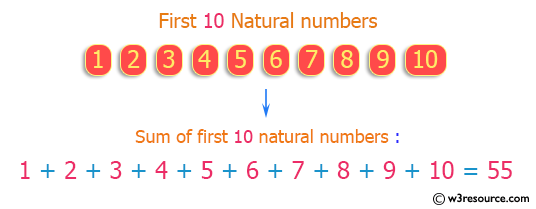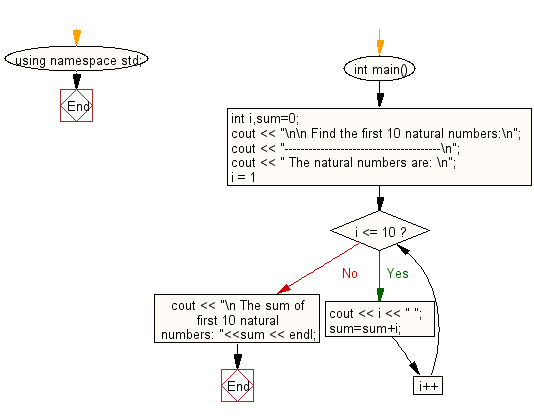﻿ C++ Exercises: Find the sum of first 10 natural numbers - w3resource# C++ Exercises: Find the sum of first 10 natural numbers

## C++ For Loop: Exercise-2 with Solution

Write a program in C++ to find the sum of first 10 natural numbers.

Pictorial Presentation:Sample Solution :-

C++ Code :

``````#include <iostream>
using namespace std;
int main()
{
int i,sum=0;
cout << "\n\n Find the first 10 natural numbers:\n";
cout << "---------------------------------------\n";
cout << " The natural numbers are: \n";
for (i = 1; i <= 10; i++)
{
cout << i << " ";
sum=sum+i;
}
cout << "\n The sum of first 10 natural numbers: "<<sum << endl;
}
``````

Sample Output:

``` Find the first 10 natural numbers:
---------------------------------------
The natural numbers are:
1 2 3 4 5 6 7 8 9 10
The sum of first 10 natural numbers: 55
```

Flowchart:C++ Code Editor:

Contribute your code and comments through Disqus.

What is the difficulty level of this exercise?

﻿

## C++ Programming: Tips of the Day

What is a smart pointer and when should I use one?

This answer is rather old, and so describes what was 'good' at the time, which was smart pointers provided by the Boost library. Since C++11, the standard library has provided sufficient smart pointers types, and so you should favour the use of std::unique_ptr, std::shared_ptr and std::weak_ptr.

There was also std::auto_ptr. It was very much like a scoped pointer, except that it also had the "special" dangerous ability to be copied - which also unexpectedly transfers ownership.

It was deprecated in C++11 and removed in C++17, so you shouldn't use it.

```std::auto_ptr<MyObject> p1 (new MyObject());
std::auto_ptr<MyObject> p2 = p1; // Copy and transfer ownership.
// p1 gets set to empty!
p2->DoSomething(); // Works.
p1->DoSomething(); // Oh oh. Hopefully raises some NULL pointer exception.
```

Ref : https://bit.ly/3mc9GHE